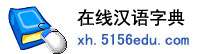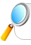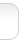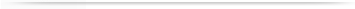汉字   按部首检索  按拼音检索雨字的男姓名 | 雨字的女姓名 | 雨开头的姓名 | 雨结尾的姓名 | 更多带雨的姓名
 雨 (男) 雨 (女) 春雨 (男) 春雨 (女) 晓雨 (女) 小雨 (女) 思雨 (女) 红雨 (男) 晓雨 (男) 小雨 (男) 红雨 (女) 洪雨 (男) 诗雨 (女) 梦雨 (女) 欣雨 (女) 时雨 (男) 庆雨 (男) 天雨 (男) 振雨 (男) 明雨 (男) 秋雨 (男) 佳雨 (女) 新雨 (男) 思雨 (男) 秋雨 (女) 建雨 (男) 雷雨 (男) 潇雨 (女) 风雨 (男) 诗雨 (男) 金雨 (男) 文雨 (男) 晨雨 (男) 德雨 (男) 丝雨 (女) 冬雨 (男) 笑雨 (女) 国雨 (男) 兆雨 (男) 晨雨 (女) 泽雨 (男) 兴雨 (男) 梦雨 (男) 心雨 (女) 文雨 (女) 志雨 (男) 潇雨 (男) 佳雨 (男) 祥雨 (男) 馨雨 (女) 华雨 (男) 长雨 (男) 鑫雨 (男) 龙雨 (男) 新雨 (女) 光雨 (男) 天雨 (女) 士雨 (男) 传雨 (男) 连雨 (男) 宏雨 (男) 中雨 (男) 星雨 (男) 东雨 (男) 夏雨 (男) 大雨 (男) 忠雨 (男) 俊雨 (男) 昌雨 (男) 笑雨 (男) 化雨 (男) 静雨 (男) 虹雨 (女) 仁雨 (男) 善雨 (男) 云雨 (男) 敬雨 (男) 田雨 (男) 书雨 (男) 时雨 (女) 孝雨 (男) 得雨 (男) 汉雨 (男) 星雨 (女) 清雨 (男) 恒雨 (男) 向雨 (男) 作雨 (男) 田雨 (女) 正雨 (男) 倩雨 (女) 雨雨 (女) 逢雨 (男) 辰雨 (男) 胜雨 (男) 顺雨 (男) 欣雨 (男) 广雨 (男) 良雨 (男) 夭雨 (男) 凤雨 (男) 沁雨 (女) 谷雨 (男) 道雨 (男) 若雨 (女) 继雨 (男) 存雨 (男) 洪雨 (女) 昕雨 (女) 成雨 (男) 甜雨 (女) 学雨 (男) 桐雨 (女) 维雨 (男) 守雨 (男) 朋雨 (男) 泽雨 (女) 冬雨 (女) 飞雨 (男) 斯雨 (女) 青雨 (男) 朝雨 (男) 树雨 (女) 霄雨 (女) 其雨 (男) 永雨 (男) 绍雨 (男) 巧雨 (女) 吉雨 (男) 清雨 (女) 保雨 (男) 嘉雨 (男) 建雨 (女) 海雨 (男) 雨雨 (男) 先雨 (男) 子雨 (男) 沁雨 (男) 世雨 (男) 翔雨 (男) 舒雨 (男) 寒雨 (男) 少雨 (男) 立雨 (男) 振雨 (女) 成雨 (女) 宗雨 (男) 静雨 (女) 肖雨 (女) 彦雨 (男) 谷雨 (女) 冰雨 (男) 金雨 (女) 夏雨 (女) 军雨 (男) 桂雨 (男) 章雨 (男) 冰雨 (女) 舒雨 (女) 筱雨 (女) 凡雨 (男) 瑞雨 (男) 会雨 (男) 尚雨 (男) 化雨 (女) 万雨 (男) 荣雨 (男) 若雨 (男) 秀雨 (男) 培雨 (男) 甘雨 (男) 知雨 (女) 震雨 (男) 明雨 (女) 鹏雨 (男) 轩雨 (女) 淑雨 (女) 喜雨 (女) 沛雨 (男) 艳雨 (女) 殿雨 (男) 恩雨 (男) 夕雨 (女) 景雨 (男) 瑞雨 (女) 虹雨 (男) 华雨 (女) 昭雨 (男) 曦雨 (女) 广雨 (女) 歆雨 (女) 启雨 (男) 兆雨 (女) 家雨 (男) 更雨 (男) 洁雨 (女) 靖雨 (女) 筱雨 (男) 扬雨 (男) 燕雨 (女) 弘雨 (男) 一雨 (男) 听雨 (女) 丰雨 (男) 方雨 (男) 元雨 (男) 平雨 (男) 夭雨 (女) 耿雨 (男) 铭雨 (男) 增雨 (男) 开雨 (男) 爱雨 (女) 霄雨 (男) 鸿雨 (男) 正雨 (女) 丙雨 (男) 泉雨 (男) 芯雨 (女) 令雨 (男) 江雨 (男) 方雨 (女) 双雨 (女) 雪雨 (男) 怀雨 (男) 贵雨 (男) 嘉雨 (女) 施雨 (女) 镇雨 (男) 帮雨 (男) 茂雨 (男) 含雨 (男) 旱雨 (男) 菡雨 (女) 圣雨 (男) 双雨 (男) 和雨 (男) 苗雨 (男) 灵雨 (女) 宝雨 (男) 银雨 (男) 克雨 (男) 友雨 (男) 纯雨 (男) 廷雨 (男) 伏雨 (男) 坤雨 (男) 俊雨 (女) 陈雨 (女) 琛雨 (女) 宵雨 (女) 晴雨 (女) 桑雨 (女) 为雨 (男) 书雨 (女) 楚雨 (男) 纪雨 (男) 辛雨 (男) 同雨 (男) 治雨 (男) 跃雨 (男) 辛雨 (女) 好雨 (女) 智雨 (男) 厚雨 (男) 伟雨 (男) 利雨 (男) 铮雨 (女) 添雨 (女) 默雨 (女) 树雨 (男) 昶雨 (女) 显雨 (男) 沐雨 (男) 风雨 (女) 非雨 (女) 班雨 (男) 露雨 (女) 蒙雨 (女) 牧雨 (女) 占雨 (男) 传雨 (女) 飘雨 (女) 孟雨 (女) 贺雨 (男) 祖雨 (男) 歆雨 (男) 丹雨 (女) 斯雨 (男) 宏雨 (女) 京雨 (男) 洁雨 (男) 吟雨 (女) 靖雨 (男) 布雨 (男) 从雨 (男) 巨雨 (男) 君雨 (女) 湘雨 (男) 灏雨 (男) 云雨 (女) 志雨 (女) 私雨 (女) 佰雨 (男) 忠雨 (女) 兰雨 (男) 宣雨 (男) 阳雨 (女) 山雨 (男) 美雨 (女) 嫣雨 (女) 婧雨 (女) 奎雨 (男) 刚雨 (男) 群雨 (男) 雷雨 (女) 君雨 (男) 鑫雨 (女) 之雨 (男) 凤雨 (女) 秀雨 (女) 梨雨 (女) 剑雨 (男) 来雨 (男) 桂雨 (女) 孝雨 (女) 沛雨 (女) 旭雨 (男) 龙雨 (女) 桐雨 (男) 好雨 (男) 召雨 (男) 恩雨 (女) 慧雨 (女) 辰雨 (女) 晗雨 (女) 登雨 (男) 常雨 (男) 本雨 (男) 高雨 (男) 韩雨 (女) 宛雨 (女) 晶雨 (女) 咏雨 (男) 净雨 (女) 中雨 (女) 叶雨 (女) 淘雨 (男) 全雨 (男) 宾雨 (男) 坚雨 (男) 遵雨 (男) 根雨 (男) 会雨 (女) 宸雨 (男) 凌雨 (女) 英雨 (男) 艳雨 (男) 枢雨 (男) 菲雨 (女) 袅雨 (男) 瀚雨 (男) 合雨 (男) 挽雨 (女) 昊雨 (男) 松雨 (男) 萌雨 (男) 忆雨 (女) 流雨 (女) 杭雨 (男) 南雨 (男) 夜雨 (男) 玲雨 (女) 露雨 (男) 舜雨 (男) 忻雨 (女) 细雨 (男) 蔚雨 (女) 梁雨 (男) 霆雨 (男) 蜀雨 (女) 福雨 (男) 兴雨 (女) 现雨 (男) 承雨 (男) 苏雨 (女) 哲雨 (男) 亭雨 (男) 婷雨 (女) 墨雨 (男) 播雨 (男) 惊雨 (男) 功雨 (女) 炳雨 (男) 圣雨 (女) 博雨 (男) 水雨 (男) 怀雨 (女) 昕雨 (男) 重雨 (男) 震雨 (女) 钧雨 (男) 浩雨 (女) 进雨 (女) 蓝雨 (女) 司雨 (女) 运雨 (男) 心雨 (男) 杰雨 (男) 玉雨 (女) 荣雨 (女) 皆雨 (女) 晋雨 (男) 西雨 (男) 蓉雨 (男) 浪雨 (男) 晗雨 (男) 舜雨 (女) 忻雨 (男) 盈雨 (女) 品雨 (男) 慕雨 (男) 泗雨 (男) 澍雨 (男) 程雨 (女) 洋雨 (男) 修雨 (男) 皆雨 (男) 少雨 (女) 仟雨 (女) 尔雨 (女) 发雨 (男) 悲雨 (男) 蓁雨 (女) 积雨 (男) 语雨 (女) 惟雨 (男) 恭雨 (男) 弘雨 (女) 及雨 (女) 衡雨 (男) 房雨 (男) 庆雨 (女) 扬雨 (女) 喜雨 (男) 宇雨 (女) 竣雨 (男) 道雨 (女) 周雨 (男) 基雨 (男) 太雨 (男) 见雨 (男) 珊雨 (女) 窗雨 (男) 换雨 (女) 送雨 (女) 获雨 (女) 婕雨 (女) 铮雨 (男) 益雨 (男) 琦雨 (女) 慕雨 (女) 加雨 (男) 夕雨 (男) 翃雨 (男) 宗雨 (女) 歌雨 (男) 宜雨 (男) 念雨 (女) 苏雨 (男) 昶雨 (男) 淼雨 (男) 竞雨 (女) 到雨 (男) 晚雨 (女) 泓雨 (男) 步雨 (男) 宽雨 (男) 祥雨 (女) 声雨 (男) 青雨 (女) 窘雨 (男) 枝雨 (男) 马雨 (男) 朝雨 (女) 澎雨 (女) 可雨 (男) 业雨 (男) 沫雨 (男) 素雨 (男) 茗雨 (女) 顺雨 (女) 后雨 (男) 捎雨 (男) 盛雨 (男) 炎雨 (男) 及雨 (男) 寿雨 (男) 芬雨 (女) 宪雨 (男) 楚雨 (女) 勤雨 (女) 亮雨 (男) 孟雨 (男) 詹雨 (男) 耕雨 (男) 生雨 (男) 观雨 (男) 禧雨 (女) 鸣雨 (女) 辉雨 (女) 源雨 (女) 伯雨 (男) 在雨 (男) 萧雨 (女) 阳雨 (男) 尧雨 (男) 丝雨 (男) 彤雨 (男) 佩雨 (女) 抒雨 (女) 四雨 (男) 官雨 (男) 陈雨 (男) 孙雨 (男) 弨雨 (男) 行雨 (男) 凭雨 (男) 彩雨 (女) 雪雨 (女) 海雨 (女) 强雨 (男) 骤雨 (男) 佃雨 (男) 湘雨 (女) 言雨 (女) 象雨 (男) 自雨 (男) 昆雨 (男) 靓雨 (女) 湛雨 (男) 石雨 (男) 美雨 (男) 涵雨 (女) 晴雨 (男) 夜雨 (女) 逢雨 (女) 载雨 (男) 号雨 (男) 琼雨 (女) 细雨 (女) 碧雨 (女) 弟雨 (男) 茜雨 (男) 郑雨 (女) 微雨 (女) 池雨 (男) 骆雨 (女) 黎雨 (男) 照雨 (男) 熙雨 (女) 撞雨 (女) 铎雨 (男) 聪雨 (男) 亦雨 (女) 杏雨 (女) 效雨 (男) 相雨 (男) 无雨 (男) 闻雨 (男) 光雨 (女) 澄雨 (男) 旭雨 (女) 宁雨 (男) 警雨 (女) 顶雨 (男) 古雨 (男) 名雨 (男) 福雨 (女) 舟雨 (女) 稼雨 (男) 玺雨 (女) 甘雨 (女) 啊雨 (男) 钲雨 (男) 如雨 (男) 开雨 (女) 季雨 (男) 柏雨 (男) 朦雨 (女) 恿雨 (女) 贝雨 (女) 施雨 (男) 宵雨 (男) 秉雨 (男) 江雨 (女) 梅雨 (男) 赤雨 (男) 绵雨 (女) 际雨 (男) 滨雨 (男) 啸雨 (男) 繁雨 (女) 嗣雨 (男) 润雨 (男) 润雨 (女) 驰雨 (男) 淑雨 (男) 姿雨 (男) 北雨 (男) 林雨 (男) 芳雨 (女) 晛雨 (女) 添雨 (男) 霁雨 (女) 奇雨 (女) 陆雨 (男) 修雨 (女) 平雨 (女) 政雨 (男) 淳雨 (男) 延雨 (男) 子雨 (女) 径雨 (男) 显雨 (女) 鉴雨 (男) 林雨 (女) 千雨 (男) 卫雨 (男) 招雨 (男) 付雨 (女) 雯雨 (女) 均雨 (男) 宝雨 (女) 远雨 (男) 战雨 (男) 乐雨 (男) 锡雨 (男) 绪雨 (女) 话雨 (女) 立雨 (女) 乃雨 (女) 白雨 (女) 升雨 (男) 泰雨 (男) 学雨 (女) 凌雨 (男) 连雨 (女) 功雨 (男) 乔雨 (女) 绵雨 (男) 航雨 (男) 示雨 (女) 坤雨 (女) 婉雨 (男) 年雨 (男) 含雨 (女) 保雨 (女) 梅雨 (女) 落雨 (女) 赐雨 (男) 磊雨 (男) 邦雨 (男) 叶雨 (男) 馨雨 (男) 伦雨 (男) 致雨 (男) 滋雨 (男) 霓雨 (男) 锦雨 (男) 沐雨 (女) 多雨 (男) 贵雨 (女)

 版权所有　在线汉语字典   浙ICP备05019169号